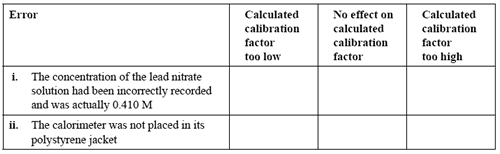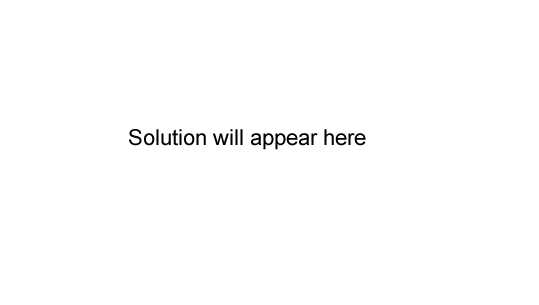Thermochemistry exercise (2006 VCE) Methanol can be produced in a reaction between carbon monoxide and hydrogen according to the following equation. CO(g) + 2H2(g) => CH3OH(g); ΔH = 90 kJ/ mol Which one of the following changes would occur when a catalyst is added to an equilibrium mixture of carbon monoxide, hydrogen and methanol? A. The value of ΔH would increase. B. The amount of methanol would increase. C. The temperature of the surroundings would increase. D. The rates of both the forward and reverse reactions would increase. Solution Which statement about the behaviour of a catalyst in this reaction is correct? A. It decreases the activation energy of the forward reaction only. B. It increases the activation energy of the forward reaction only. C. It decreases the activation energies of both the forward and back reactions. D. It increases the activation energies of both the forward and back reactions. SolutionConsider the following information for the reaction A + B → C. heat of reaction -120 kJ/ mol activation energy +200 kJ/ mol The activation energy, in kJ /mol, for the reaction C → A + B, is A. -320 B.- 80 C. +80 D. +320 SolutionThe calibration factor of a calorimeter can be determined by performing in the calorimeter a reaction which produces a known quantity of energy, and measuring the rise in temperature. In one experiment, 50.0 mL of 0.400 M lead nitrate was added to 50.0 mL of 0.760 M potassium iodide in a solution calorimeter encased in a polystyrene insulating jacket. The mixture was stirred continuously and the temperature rose from 18.42°C to 20.50°C as the following reaction occurred. Pb(NO3)2(aq) + 2KI(aq) → PbI2(s) + 2KNO3(aq); ΔH = -49.0 kJ mol-1 a.Calculate the amount, in mole, of lead nitrate. Solution b. Calculate the amount, in mole, of potassium iodide. Solution c. Calculate the energy released by the reaction in the calorimeter, in J. Solution d. Calculate, to an appropriate number of significant figures, the calibration factor of the calorimeter and its contents, in J°C-1 . Solution Two errors that might have occurred during this experiment are listed below. For each error, indicate the likely effect on the calculated calorimeter factor by placing a tick in the appropriate box.Solution Give an explanation for your answer to part ii. above. Solution